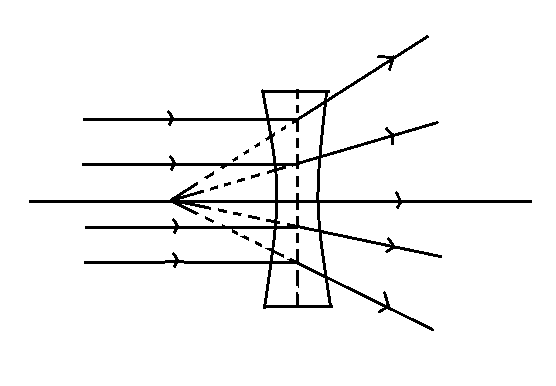# What is a diverging lens also known as?A. PositiveB. NegativeC. Neutral D. None

Last updated date: 30th Jan 2023
Total views: 238.5k
Views today: 5.32kVerified
238.5k+ views
Hint: In order to solve this question, firstly we will understand the concept of diverging lens i.e. It directs light away from the optical center or axis of the lens. Then we will compare the given options to find the required answer.The diverging lens directs light away from the optical center or axis of the lens. The diverging lens is also known as negative lens, concave lens or dispersive lens. Diverging lenses are available in different shapes, but all diverging lenses are the negative sign signifies that the image is virtual.
A lens is a transmissive optical device that affects the focus of a light beam through refraction. The distance from this point to the lens is also known as the focal length, though it is negative. Convex-concave lenses can be either positive or negative, depending on the relative curvatures of the two surfaces.
In a concave lens, spherical surfaces are recessed inwards. It is thinned out in the middle.
A diverging lens that causes parallel light rays to spread out – hence diverge – away from the optical axis once exiting the lens. The lens surfaces may be plano-concave, double-concave or concave-convex. The edge of a diverging lens is always thicker than the center. Parallel light rays entering a diverging lens to form virtual images of the object that light ray is originating from.
Therefore, we conclude that a diverging lens is also known as a negative lens.

Hence, option B is correct.

Note:
While solving this question, we should also know that diverging lenses are used in binoculars, telescopes, glasses, cameras, flashlights etc. Also one must know that a lens with one of its sides converging and the other diverging is known as a meniscus lens.